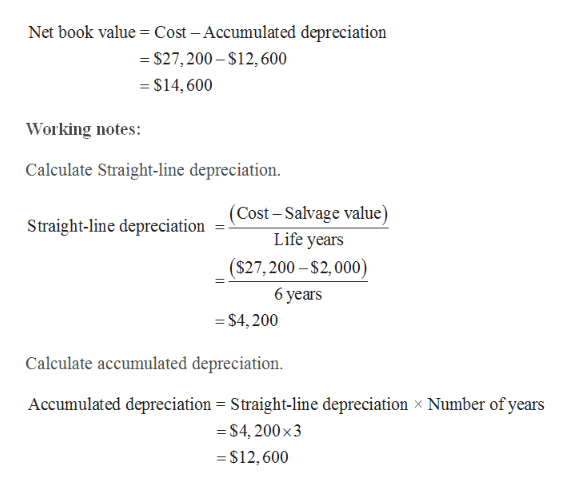# Computing Depreciation, asset book value and gain or loss on asset sale Palepu Company owns and operates a delivery van that originally cost \$27,200. Straight-line depreciation on the van has been recorded for 3 years, with a \$2,000 expected salvage value at the end of its estimated 6 years useful life. Depreciation was last recorded at the end of the third year, at which time Palepu disposes of this van. a. Compute the net book value of the van on the sale date.b. Compute the gain or loss on sale of the van if its sales price is for:1. Cash equal to book value of van2. \$15,000 cash3. \$12,000 cash

Question
38 views

Computing Depreciation, asset book value and gain or loss on asset sale
Palepu Company owns and operates a delivery van that originally cost \$27,200.
Straight-line depreciation on the van has been recorded for 3 years, with a \$2,000 expected salvage value at the end of its estimated 6 years useful life. Depreciation was last recorded at the end of the third year, at which time Palepu disposes of this van.
a. Compute the net book value of the van on the sale date.
b. Compute the gain or loss on sale of the van if its sales price is for:
1. Cash equal to book value of van
2. \$15,000 cash
3. \$12,000 cash

check_circle

Step 1

a.

Calculate the net book value of t...help_outlineImage TranscriptioncloseNet book value Cost -Accumulated depreciation = \$27,200-S12,600 = \$14,600 Working notes: Calculate Straight-line depreciation. |(Cost-Salvage value) Life years Straight-line depreciation (S27,200-\$2,000) 6 years =\$4,200 Calculate accumulated depreciation Accumulated depreciation = Straight-line depreciation x Number of years =S4, 200 x3 \$12,600 fullscreen

### Want to see the full answer?

See Solution

#### Want to see this answer and more?

Solutions are written by subject experts who are available 24/7. Questions are typically answered within 1 hour.*

See Solution
*Response times may vary by subject and question.
Tagged in